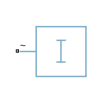Since R2021a

•Libraries:
Simscape / Electrical / Passive

## Description

The Constant Current Load (Three-Phase) block implements a constant current load for a three-phase supply.

This block consumes a current equal to the value of the Consumed current (RMS) parameter as long as the voltage from the three-phase supply is equal to or greater than the value specified for the Minimum supply voltage (phase-to-phase RMS) parameter.

When the voltage from the three-phase supply drops below the value of Minimum supply voltage (phase-to-phase RMS), the load behaviour changes and the block models a load with constant resistance.

### Equations

If the three-phase voltages are balanced, the phase-to-phase RMS voltage is defined by this equation:

`${V}_{lin{e}_{RMS}}=\sqrt{{\left({v}_{a}-{v}_{dc}\right)}^{2}+{\left({v}_{b}-{v}_{dc}\right)}^{2}+{\left({v}_{c}-{v}_{dc}\right)}^{2}},$`

where ${v}_{dc}=\frac{{v}_{a}+{v}_{b}+{v}_{c}}{3}.$

The currents for each of the three phases are defined by this equation:

`${i}_{k}\left(t\right)=\frac{{v}_{k}\left(t\right)}{{R}_{equiv}},$`

where Requiv is the equivalent resistance and depends on value of the Minimum supply voltage (phase-to-phase RMS) parameter.

If the voltage from the three-phase supply is greater than the value specified for the Minimum supply voltage (phase-to-phase RMS) parameter, the equivalent resistance is defined by:

`${R}_{equiv}=\frac{\frac{{v}_{pk}}{\sqrt{2}}}{{I}_{RM{S}_{consumed}}},$`

where vpk is the voltage peak magnitude and IRMSconsumed is the value of the Consumed current (RMS) parameter.

If the voltage from the three-phase supply is less than the value specified for the Minimum supply voltage (phase-to-phase RMS) parameter, the equivalent resistance is defined by:

`${R}_{equiv}=\frac{{v}_{phRM{S}_{min}}}{{I}_{RM{S}_{consumed}}},$`

where ${v}_{phRM{S}_{min}}=\frac{{v}_{lineRM{S}_{min}}}{\sqrt{3}}$ is the phase-to-ground RMS minimum voltage.

### Faults

The Constant Current Load (Three-Phase) block allows you to model an electrical fault as an open circuit. The block can trigger fault events either:

• At a specific time

• When a power limit is exceeded for longer than a specific time interval

You can also choose whether to issue an assertion when a fault occurs by using the Reporting when a fault occurs parameter. The assertion can take the form of a warning or an error. By default, the block does not issue an assertion.

If the block is in a network that is compatible with frequency-time simulation mode, you can perform a load-flow analysis on the network. A load-flow analysis provides steady-state values that you can use to initialize a machine.

For more information, see Perform a Load-Flow Analysis Using Simscape Electrical and Frequency and Time Simulation Mode.

## Ports

### Conserving

expand all

Expandable three-phase electrical port associated with the three-phase voltage.

## Parameters

expand all

Whether to model composite or expanded three-phase ports.

Composite three-phase ports represent three individual electrical conserving ports with a single block port. You can use composite three-phase ports to build models that correspond to single-line diagrams of three-phase electrical systems.

Expanded three-phase ports represent the individual phases of a three-phase system using three separate electrical conserving ports.

### Main

Root mean square current consumed by the block.

Minimum voltage, in phase-to-phase RMS, that the supply must provide for the block to act as a current load.

### Faults

Select `On` to enable faults modeling and expose parameters that allow you to select the reporting method and specify the trigger mechanism.

Conductance during the open-circuit state.

#### Dependencies

To enable this parameter, set Enable open-circuit fault to `On`.

Choose whether to issue an assertion when a fault occurs:

• `None` — The block does not issue an assertion.

• `Warn` — The block issues a warning.

• `Error` — Simulation stops with an error.

#### Dependencies

To enable this parameter, set Enable open-circuit fault to `On`.

Whether to trigger the fault based on temporal or behavioral conditions.

#### Dependencies

To enable this parameter, set Enable open-circuit fault to `On`.

Simulation time when the block enters the faulted state.

#### Dependencies

To enable this parameter, set Enable open-circuit fault to `On` and Fault trigger to `Temporal`.

Maximum permissible power value. If the power exceeds this value for longer than the Time to fail when exceeding power parameter value, then the block enters the faulted state.

#### Dependencies

To enable this parameter, set Enable open-circuit fault to `On` and Fault trigger to `Behavioral`.

Maximum length of time that the power can exceed the maximum permissible value without triggering the fault.

#### Dependencies

To enable this parameter, set Enable open-circuit fault to `On` and Fault trigger to `Behavioral`.

## Version History

Introduced in R2021a